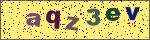semver的文档

https://docs.npmjs.com/cli/v6/using-npm/semver

Tilde Ranges ~1.2.3 ~1.2 ~1
Allows patch-level changes if a minor version is specified on the comparator. Allows minor-level changes if not.

~1.2.3 := >=1.2.3 <1.(2+1).0 := >=1.2.3 <1.3.0
~1.2 := >=1.2.0 <1.(2+1).0 := >=1.2.0 <1.3.0 (Same as 1.2.x)
~1 := >=1.0.0 <(1+1).0.0 := >=1.0.0 <2.0.0 (Same as 1.x)
~0.2.3 := >=0.2.3 <0.(2+1).0 := >=0.2.3 <0.3.0
~0.2 := >=0.2.0 <0.(2+1).0 := >=0.2.0 <0.3.0 (Same as 0.2.x)
~0 := >=0.0.0 <(0+1).0.0 := >=0.0.0 <1.0.0 (Same as 0.x)
~1.2.3-beta.2 := >=1.2.3-beta.2 <1.3.0 Note that prereleases in the 1.2.3 version will be allowed, if they are greater than or equal to beta.2. So, 1.2.3-beta.4 would be allowed, but 1.2.4-beta.2 would not, because it is a prerelease of a different [major, minor, patch] tuple.
Caret Ranges ^1.2.3 ^0.2.5 ^0.0.4
Allows changes that do not modify the left-most non-zero digit in the [major, minor, patch] tuple. In other words, this allows patch and minor updates for versions 1.0.0 and above, patch updates for versions 0.X >=0.1.0, and no updates for versions 0.0.X.

Many authors treat a 0.x version as if the x were the major "breaking-change" indicator.

Caret ranges are ideal when an author may make breaking changes between 0.2.4 and 0.3.0 releases, which is a common practice. However, it presumes that there will not be breaking changes between 0.2.4 and 0.2.5. It allows for changes that are presumed to be additive (but non-breaking), according to commonly observed practices.

^1.2.3 := >=1.2.3 <2.0.0
^0.2.3 := >=0.2.3 <0.3.0
^0.0.3 := >=0.0.3 <0.0.4
^1.2.3-beta.2 := >=1.2.3-beta.2 <2.0.0 Note that prereleases in the 1.2.3 version will be allowed, if they are greater than or equal to beta.2. So, 1.2.3-beta.4 would be allowed, but 1.2.4-beta.2 would not, because it is a prerelease of a different [major, minor, patch] tuple.
^0.0.3-beta := >=0.0.3-beta <0.0.4 Note that prereleases in the 0.0.3 version only will be allowed, if they are greater than or equal to beta. So, 0.0.3-pr.2 would be allowed.
When parsing caret ranges, a missing patch value desugars to the number 0, but will allow flexibility within that value, even if the major and minor versions are both 0.

^1.2.x := >=1.2.0 <2.0.0
^0.0.x := >=0.0.0 <0.1.0
^0.0 := >=0.0.0 <0.1.0
A missing minor and patch values will desugar to zero, but also allow flexibility within those values, even if the major version is zero.

^1.x := >=1.0.0 <2.0.0
^0.x := >=0.0.0 <1.0.0
Range Grammar
Putting all this together, here is a Backus-Naur grammar for ranges, for the benefit of parser authors:

range-set  ::= range ( logical-or range ) *
logical-or ::= ( ' ' ) * '||' ( ' ' ) *
range      ::= hyphen | simple ( ' ' simple ) * | ''
hyphen     ::= partial ' - ' partial
simple     ::= primitive | partial | tilde | caret
primitive  ::= ( '<' | '>' | '>=' | '<=' | '=' ) partial
partial    ::= xr ( '.' xr ( '.' xr qualifier ? )? )?
xr         ::= 'x' | 'X' | '*' | nr
nr         ::= '0' | ['1'-'9'] ( ['0'-'9'] ) *
tilde      ::= '~' partial
caret      ::= '^' partial
qualifier  ::= ( '-' pre )? ( '+' build )?
pre        ::= parts
build      ::= parts
parts      ::= part ( '.' part ) *
part       ::= nr | [-0-9A-Za-z]+

发表评论